# Function List

Required functions

version3

Print message functions

Transform functions

getTransformMatrix

Draw functions

Primitives

Attribute controls

setDepth

Lights

Camera function

Stroke details

Dot details

Output details

Texture operations

newTex, delTex, getSize, swapTex, drawTo, castTex, blendTex, copyTex, savePNG, loadPNG, saveEXR, loadEXR, rotateTex, flipTex, resizeTex, trimTex

Utility tools

rgb2hsl,hsl2rgb,rgb2hsv,hsv2rgb,rgb2cmy,cmy2rgb,rgb2cmyk,cmyk2rgb,cmy2cmyk,cmyk2cmy,hsl2hsv,hsv2hsl

Run code functions

# Detailed descriptions

## version3

version3() function must be called in the first line. It put all functions in the table pw3 to the global field. If you don't call it, you need to add a pw3. prefix to all the functions provided by PixelsWorld. For instance, the following demonstrated function print() should be pw3.print(). On the contrary, If you call the function version3() in the first line, the pw3. prefix could be omitted. This function is designed for backward compatibility. We recommend calling version3() in the first line any time you use Lua render mode.

version3.lua

version3()
println("Hello PixelsWorld! ")


without_version3.lua

pw3.println("Hello PixelsWorld! ")


## print

print(str),print(str,brightness),print(str,r,g,b),print(str,r,g,b,a) prints message to the left top corner of your scene.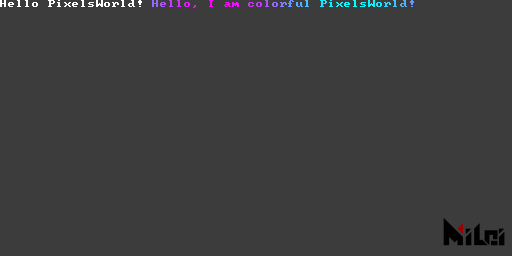print.lua

version3()
print("Hello PixelsWorld! ")
str = "Hello, I am colorful PixelsWorld! "
for i=1,#str do
local c = str:sub(i,i)
local phase = math.sin(i/#str*TPI + time*10) / 2 + .5
print(c,phase,1-phase,1,1)
end


## println

println(str),println(str,brightness),println(str,r,g,b),println(str,r,g,b,a) prints message to the left top corner of your scene, this function adds \n in the end of input string.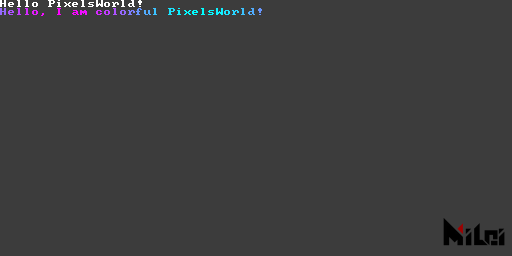println.lua

version3()
println("Hello PixelsWorld! ")
str = "Hello, I am colorful PixelsWorld! "
for i=1,#str do
local c = str:sub(i,i)
local phase = math.sin(i/#str*TPI + time*10) / 2 + .5
-- println(c,phase,1-phase,1,1)
print(c,phase,1-phase,1,1)
end


alert(str) prints caution message, equals to println(str,1,1,0,1)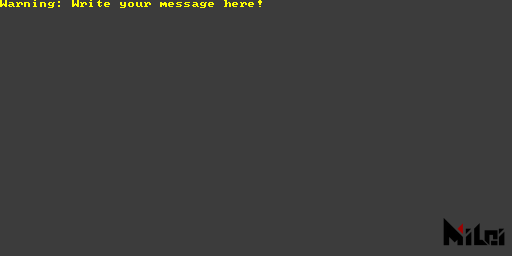version3()


## move

move(x,y),move(x,y,z)moves Paintbrush.

All transforms are done basing on the Paintbrush coordinate.

Example: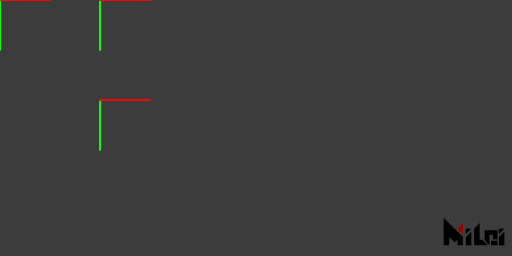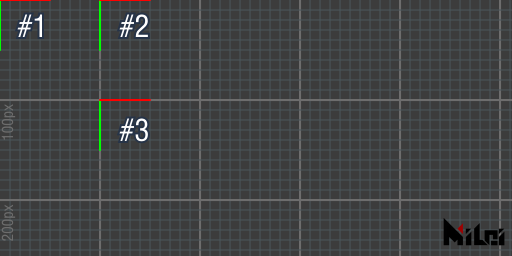move.md

version3()
coord() -- show Paintbrush coordinate
move(100,0)
coord() -- show Paintbrush coordinate
move(0,100)
coord() -- show Paintbrush coordinate


Finally, the Paintbrush is located at (100,100,0).

## scale

scale(ratio),scale(x,y),scale(x,y,z)scales the Paintbrush coordinate.

All transforms are done basing on the Paintbrush coordinate.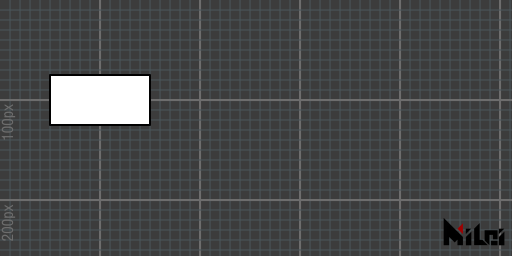scale.lua

version3()
move(100,100)
scale(2)
rect(50,25)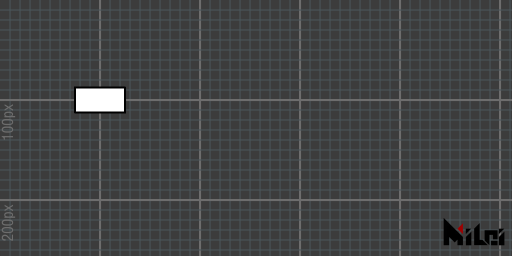no_scale.lua

version3()
move(100,100)
--scale(2)
rect(50,25)


## rotateZ

rotate(theta)rotates Paintbrush with theta radians. rotateX(theta) rotates Paintbrush along X axis. rotate(theta) equals to rotateZ(theta).

All transforms are done basing on the Paintbrush coordinate.

• If you are not familiar with radians, use d2r(degree) to convert a degree to a radian. For example：rotate(d2r(90)) means rotate 90 degrees.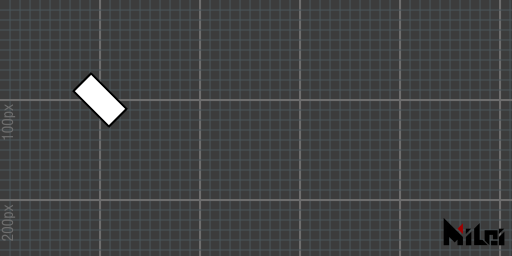rotate_degree.lua

version3()
move(100,100)
rotate(d2r(45))
rect(50,25)version3()
move(100,100)
rotate(PI/4)
rect(50,25)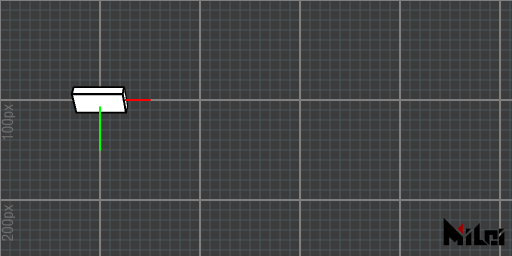rotateX.lua

version3()
dim3()
move(100,100)
grid()
coord()
rotateX(d2r(45))
cube(50,25,10)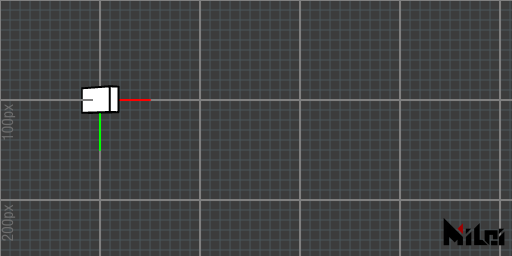rotateY.lua

version3()
dim3()
move(100,100)
grid()
coord()
rotateY(d2r(45))
cube(50,25,10)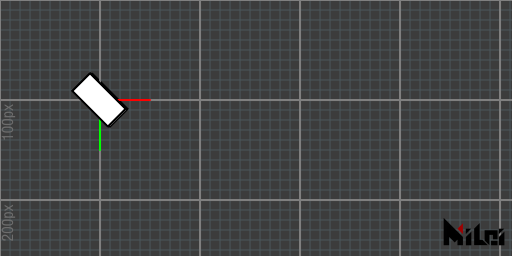rotateZ.lua

version3()
dim3()
move(100,100)
grid()
coord()
rotateZ(d2r(45))
cube(50,25,10)


## twirl

twirl(theta,x,y,z) rotates Paintbrush along (x,y,z) axis with theta radians. For example, above-mentioned rotateX(theta) equals to twirl(theta,1,0,0).

All transforms are done basing on the Paintbrush coordinate.

• twirl is an matrix implementation of quaternion rotation.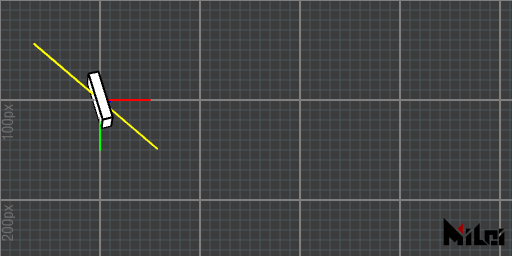twirl.lua

version3()
dim3()
move(100,100)
grid()
coord()
stroke(1,1,0)
line(-50,-50,-50,50,50,50)
stroke(0,0,0)
twirl(d2r(90),1,1,1)
cube(50,25,10)


## endGroup

1. beginGroup(),endGroup() creates a children transformation group. Transformations(move,scale,rotate,twirl) between beginGroup() and endGroup() will be canceled after calling endGroup().
2. beginGroup(mat) pushes mat as a children transformation group (Use getTransformMatrix to get the transform matrix).

It equals to pushMatrix() and popMatrix() in Processing.

Example: The following two code are equivalent.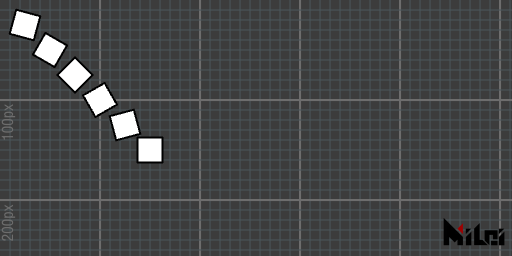group.lua

version3()
for i = 1,6 do
beginGroup()
move(i*25,i*25)
rotate(d2r(15*i))
rect(25)
endGroup()
endwithout_group.lua

version3()
for i = 1,6 do
move(i*25,i*25)
rotate(d2r(15*i))
rect(25)
rotate(d2r(-15*i))
move(-i*25,-i*25)
end


## endGlobal

Draw functions between beginGlobal and endGlobal will draw shapes in global coordinates.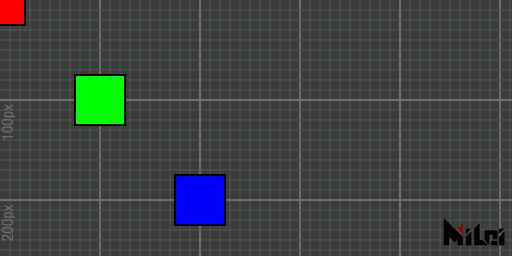beginGlobal.lua

version3()
move(100,100)
fill(0,1,0) -- green
rect(50) -- Dran on (100,100,0)
beginGlobal()
fill(1,0,0) -- red
rect(50) -- Draw on (0,0,0)
endGlobal()
move(100,100)
fill(0,0,1) -- blue
rect(50) -- Draw on (200,200,0)


## global2local

global2local(x,y,z) converts a global point to a local point. Returns 3 doubles.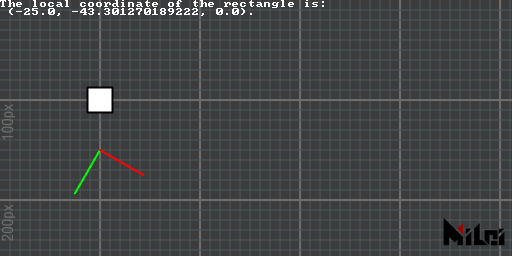global2local.lua

version3()
move(100,100)
rect(25)
move(0,50)
rotate(d2r(30))
coord()
x,y,z=global2local(100,100,0)
println("The local coordinate of the rectangle is:\n (" .. x .. ", " .. y .. ", " .. z .. ").")


## local2global

local2global(x,y,z) converts a local point to a global point. Returns 3 doubles.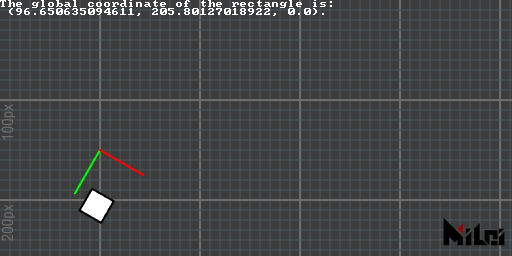local2global.lua

version3()
move(100,100)
move(0,50)
rotate(d2r(30))
coord()
move(25,50)
rect(25)
x,y,z=local2global(0,0,0)
println("The global coordinate of the rectangle is:\n (" .. x .. ", " .. y .. ", " .. z .. ").")


## global2screen

global2screen(x,y,z) converts a global point to a screen point. Returns 3 doubles.

The result is affected by perspective mode.global2screen.lua

version3()
dim3()
move(100,100,0)
move(0,50,0)
rotateZ(d2r(30))
coord()
move(25,50,0)
cube(25)
x,y,z=global2screen(local2global(0,0,0))
println("The screen coordinate of the rectangle is:\n (" .. x .. ", " .. y .. ", " .. z .. ").")


## screen2global

screen2global(x,y,z) converts a screen point to a global point. Returns 3 doubles.

The result is affected by perspective mode.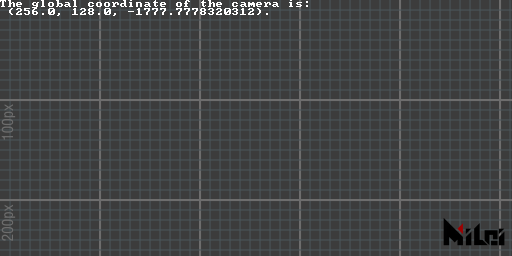screen2global.lua

version3()
dim3()

x,y,z=screen2global(0,0,0)
println("The global coordinate of the camera is:\n (" .. x .. ", " .. y .. ", " .. z .. ").")


## getTransformMatrix

getTransformMatrix() returns a column major 4x4 transform matrix.

mat[i][j] returns the entry in ith column jth row. (i,jrange: 1~4)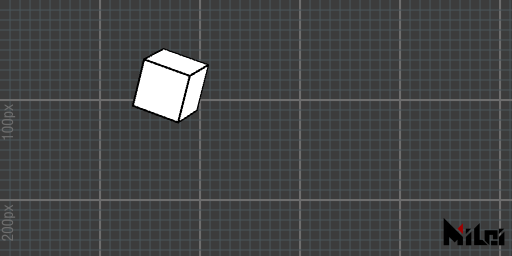matrix.lua

version3()
dim3()

beginGroup()
move(width/3,height/3)
twirl(d2r(30),1,1,1)
cubetransform = getTransformMatrix()
endGroup()

beginGroup(cubetransform)
cube(50)
endGroup()


## tri

1. tri(radius) draws a regular triangle with radius radius, and the triangle will point to the positive direction of the Y-axis.
2. tri() equals to tri(100)
3. tri(w,h)draws a triangle with base length w and height h.
4. tri(p1x,p1y,p2x,p2y,p3x,p3y) draws a triangle basing on 3 points p1,p2,p3.
5. tri(p1x,p1y,p1z,p2x,p2y,p2z,p3x,p3y,p3z) draws a triangle basing on 3 points p1,p2,p3.
• Add dim3() after version3(), and add a camera layer to your Ae comp to view the 3D triangle.
• The first 3 functions set normals to (0,0,-1) basing on the current Paintbrush coordinate. The last 2 functions calculate normals with formula: cross(p1-p2,p3-p2).
• tri is an abbreviation of triangle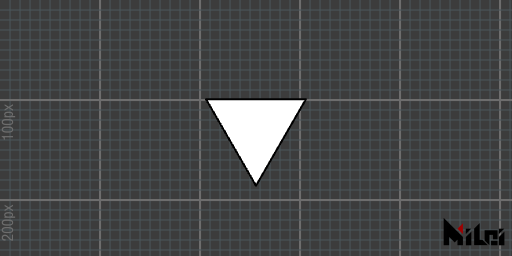tri1.lua

version3()
move(width/2,height/2)
tri(100)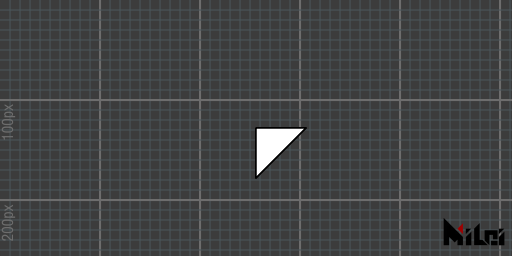tri5.lua

version3()
dim3()
move(width/2,height/2)
tri(0,0,100,50,0,0,0,50,0)


1. quad(p1x,p1y,p2x,p2y,p3x,p3y,p4x,p4y)
2. quad(p1x,p1y,p1z,p2x,p2y,p2z,p3x,p3y,p3z,p4x,p4y,p4z)
• Draws 2 triangles with order: p1,p2,p3,then p1,p3,p4.version3()
move(200,100)


## rect

1. rect(size) draws a square with size.
2. rect() equals to rect(100)
3. rect(width,height) draws a rectangle with width width and height height.
• The intersection point of the rectangle is at the origin of the Paintbrush coordinate.
• rect is an abbreviation of rectangle.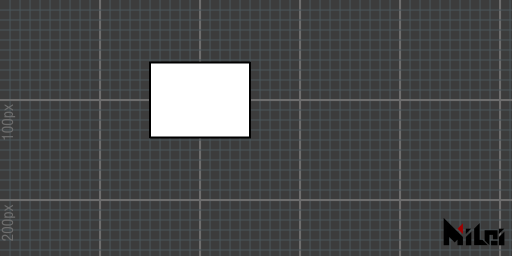rect.lua

version3()
move(200,100)
rect(100,75)


## circle

1. circle(radius) draws a circle with radius radius.
2. circle() equals to circle(100)
3. circle(radius, div) draws a circle with radius radius, and subdivision div.

Default subdivision: 128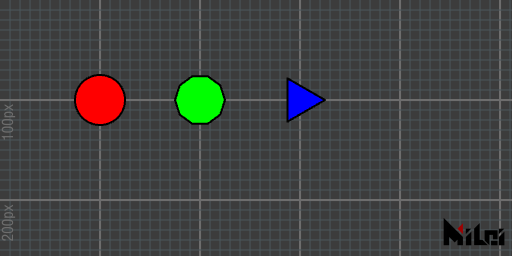circle.lua

version3()

move(100,100)
fill(1,0,0)
circle(25)

move(100,0)
fill(0,1,0)
circle(25,10)

move(100,0)
fill(0,0,1)
circle(25,3)


## ellipse

1. ellipse(radiusx,radiusy)draws an ellipse with x radiusradiusx, y radius radiusy.
2. ellipse() equals to ellipse(100,100)
3. ellipse(radiusx,radiusy,div) draws an ellipse with x radiusradiusx, y radius radiusy and subdivision div.

Default subdivision: 128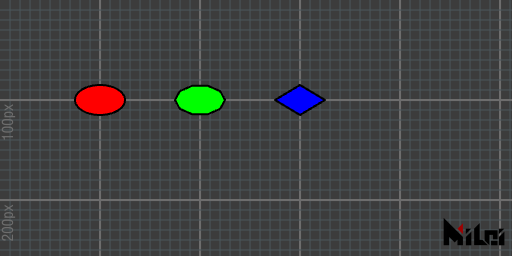ellipse.lua

version3()

move(100,100)
fill(1,0,0)
ellipse(25,15)

move(100,0)
fill(0,1,0)
ellipse(25,15,10)

move(100,0)
fill(0,0,1)
ellipse(25,15,4)


## line

1. line(p1x,p1y,p2x,p2y) draws a line.
2. line(p1x,p1y,p1z,p2x,p2y,p2z) draws a 3d line.
3. line() equals to line(0,0,0,100,100,100)
• Use stroke(r,g,b) to change the color.
• UsestrokeWidth(width) to change the width.
• Use noStroke() to turn off line render, stroke() to turn on line render.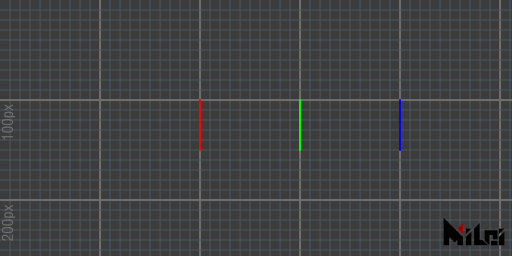line.lua

version3()

move(200,100,0)
stroke(1,0,0)
line(0,0,0,50)

move(100,0,0)
stroke(0,1,0)
line(0,0,0,50)

move(100,0,0)
stroke(0,0,1)
line(0,0,0,50)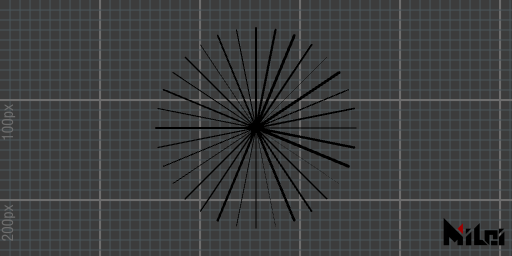line_circle.lua

version3()
math.randomseed(1)
num = 32

move(width/2,height/2)

for i=1,num do
beginGroup()
rotateZ(d2r(360/num*i))
strokeWidth(math.random()*3)
line(0,0,100,0)
endGroup()
end


## par

1. par(x) draws a point at(x,0,0).
2. par(x,y) draws a point at(x,y,0).
3. par(x,y,z) draws a point at(x,y,z).
4. par() equals to par(0,0,0)
• By default, the point rendering is turned off, use dot() to turn it on. You can call noDot() to turn it off again.
• dotRadius(radius) controls point radius.
• dot(r,g,b) controls point color.
• dotGlobal() makes point be avoid to be squeezed by scale. By default, points are rendered with dotLocal().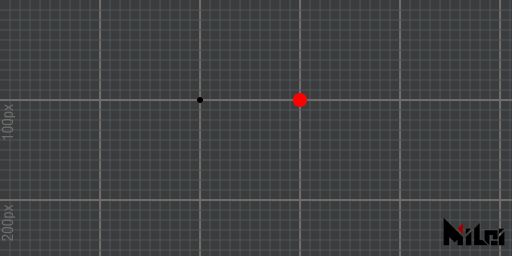par.lua

version3()

dot()
move(200,100)
par()

dot(1,0,0)
move(100,0)
par()


## cube

1. cube(size) draws a cube with size size
2. cube(sizex,sizey,sizez) draws a cuboid with dimension sizex,sizey,sizez.
3. cube() equals to cube(100)
4. cube()

Negative inputs or negative scale() would cause wrong normals.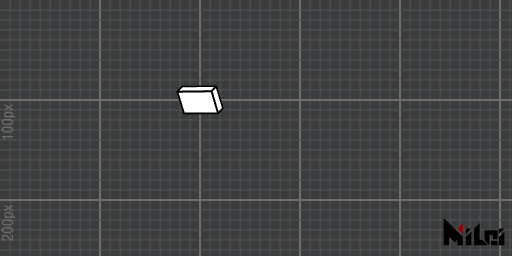cube.lua

version3()
dim3()

move(200,100,0)
rotateY(d2r(30))
rotateX(d2r(30))
cube(40,25,10)


## tet

1. tet(radius) draws a regular tetrahedron with radius radius.
2. tet() equals to tet(50)
3. tet(p1x,p1y,p1z,p2x,p2y,p2z,p3x,p3y,p3z,p4x,p4y,p4z) draws tetrahedron basing on 4 points p1,p2,p3,p4.
• The 3rd function generates normals basing on tri function and calls it with the following points order: p1,p2,p3; p2,p1,p4; p3,p2,p4; p1,p3,p4.. See also: tri.
• tet is an abbreviation of tetrahedron.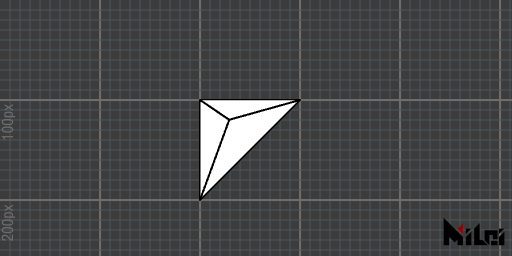tet.lua

version3()
dim3()

move(200,100,0)
tet(0,0,0,
100,0,0,
0,100,0,
30,20,-50
)


## cone

1. cone(size) draws a cone with base circle radius size and height 2*size.
2. cone() equals to cone(50).
3. cone(radius,height) draws a cone with base circle radius radius and height height.
4. cone(radius,height,div) draws a cone with base circle radius radius, height height and subdivision div.
• Default subdivision: 64
• If sub is greater than 16, the sidestroke and base point will be hidden. Negative inputs or negative scale() would cause wrong normals.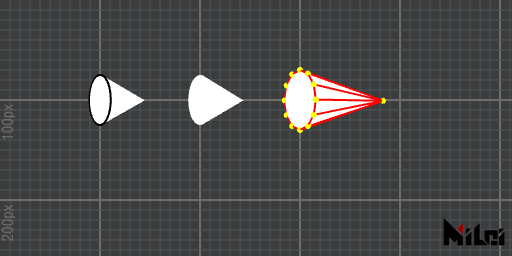cone.lua

version3()
dim3()
move(100,100,0)

beginGroup()
rotateY(d2r(60))
cone(25)
endGroup()

move(100,0,0)
beginGroup()
rotateY(d2r(60))
noStroke()
cone(25)
endGroup()

move(100,0,0)
beginGroup()
rotateY(d2r(60))
stroke(1,0,0)
dot(1,1,0)
cone(30,100,12)
endGroup()


## ball

1. ball(radius) draws a sphere with radius radius.
2. ball() equals to ball(50).
3. ball(radius,level) draw a ball with radius radius and subdivision level level.
• Default subdivision level 4.
• level must be non-negative.
• level 0 generates a regular octahedron.
• If level is greater than 2, the strokes and points will be hidden. Negative inputs or negative scale() would cause wrong normals.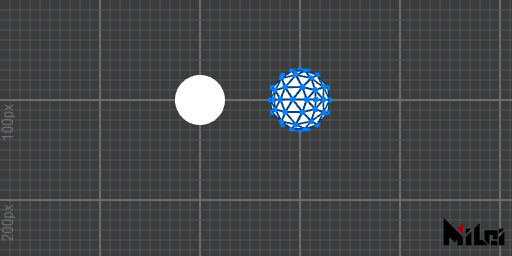ball.lua

version3()
dim3()
move(200,100,0)

beginGroup()
rotateY(d2r(60))
ball(25)
endGroup()

move(100,0,0)
beginGroup()
rotateY(d2r(60))
stroke(0,0.25,0.5)
dot(0,0.5,1)
ball(30,2)
endGroup()


## tube

1. tube(size) draws a tube with base circle radius size and height 2*size.
2. tube() equals to tube(50)
3. tube(radius,height)draws a tube with base circle radius radius and height height.
4. tube(radius1,radius2,height) draws a tube with close base circle radius radius1, far base circle radius radius2 and height height.
5. tube(radius1,radius2,height,div) can change the subdivision.
6. tube(radius1,radius2,height,div,needMesh) can change render 2 base circles or not.
7. tube(radius1,radius2,height,div,needMesh1,needMesh2) can change render 2 base circles or not separately.
• Default div: 64.
• needMesh is true by default.
• If div is greater than 16, the side strokes and the base points will be hidden. Negative inputs or negative scale() would cause wrong normals.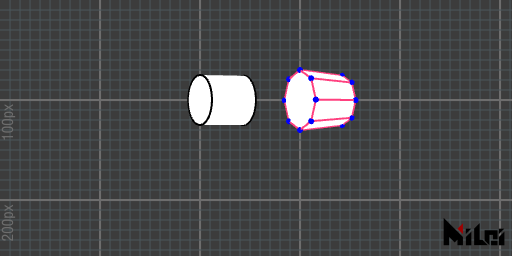tube.lua

version3()
dim3()
move(200,100,0)

beginGroup()
rotateY(d2r(60))
tube(25)
endGroup()

move(100,0,0)
beginGroup()
rotateY(d2r(60))
stroke(1,0.25,0.5)
dot(0,0,1)
tube(30,25,50,8)
endGroup()


## image

1. image(id,width,height) draws an image with width width and height height.
• id is texture id,PARAM0~PARAM9means texture load from the layer parameters, INPUT means input image, OUTPUT means output image.
• If id is OUTPUT, it will be slow because we need to take a screenshot of your scene.
• The difference with in2out(id) is, image(id, width, height) draws a rectangle with a texture, which could interact with the depth buffers, where in2out(id) just copy pixels from one texture to another. This also means the image that is drawn to the screen is inverted by default (The default coordinate system of Ae is Y axis downward), so you may need rotateX(PI) to fix it. (And we don't recommend calling scale(1,-1), which may cause wrong normals problem.

Example: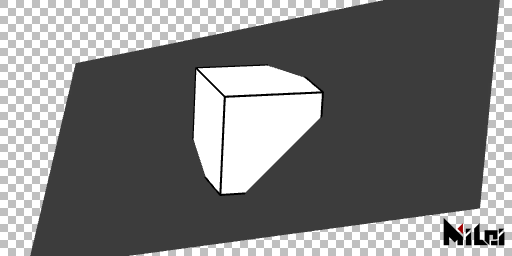render_image.lua

version3()
dim3()
move(width/2,height/2)
twirl(d2r(45),-1,1,0)
beginGroup()
rotateX(d2r(180))
image(INPUT,width,height)
endGroup()
twirl(d2r(60),1,1,1)
cube()


## imageAlign

New in v3.3.0

imageAlign(rule) changes the image orientation rendered by image function. The syntax of rule is as follows

• rule is a 4-length-string.
• 1st char should be + or -
• 2nd char should be one of x,y,z
• 3rd char should be + or -
• 4th char should be one of x,y,z

rule represents which painter coordinates the texture UV coordinate (the origin is left bottom corner) should align with. By default, the rule is "+x+y", it represents u aligns with positive x, v aligns with positive y.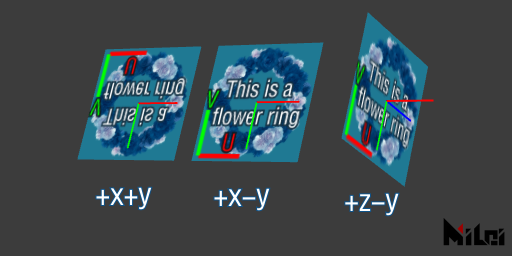imageAlign.lua

version3()
dim3()

move(100,100,0)
coord()
image(PARAM0,128,128)

move(150,0,0)
coord()
imageAlign("+x-y")
image(PARAM0,128,128)

move(150,0,0)
coord()
imageAlign("+z-y")
image(PARAM0,128,128)


## imageAnchor

New in v3.5.0.

• imageAnchor(u,v) specifies the uv position of the anchor point of the image.
• imageAnchor(a) is a shortcut of imageAnchor(a,a)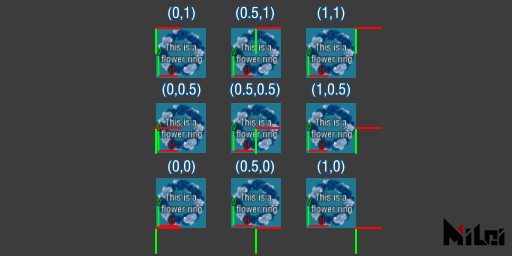## text

New in v3.5.0

text(str) renders text onto the screen.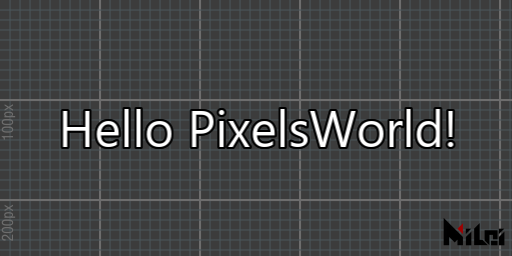text.lua

version3()
move(width/2,height/2)
textAlign("+x-y")
text("Hello PixelsWorld!")


By the way, the following functions can control the style of the text.

## textSize

New in v3.5.0

• textSize(size,resolution) set the text size to be rendered. size controls the size of the text, resolution controls the resolution of the text.
• textSize(size) equals to textSize(size,size)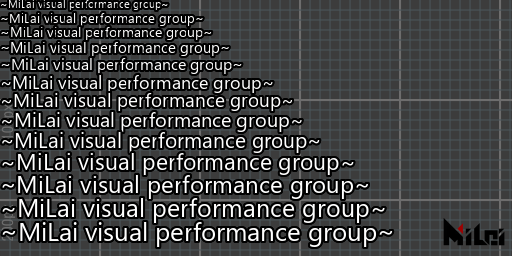textSize.lua

version3()
textAlign("+x-y")
textAnchor(0,1)
for i=1,13 do
local sz = i  + 8
textSize(sz)
text("~MiLai visual performance group~")
move(0,sz + 4)
end


## textFont

New in v3.5.0

• textFont(fontFileName) set the font of the text to be rendered. fontFileName is the font-file's name located in C:\Windows\Fonts. (Right click the file, click Properties, you would see the font-file's name. E.g.: textFont("arial.ttf"))。

You can also use the full path of a font. Yes, you can load font files in everywhere. (textFont([[D:\MyFolder\arial.ttf]]))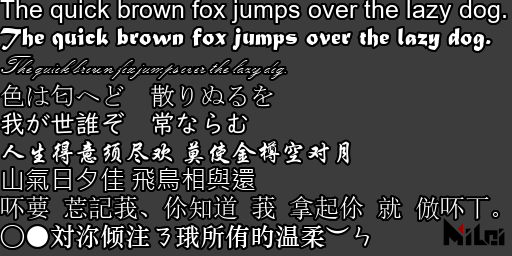textFont.lua

version3()

textAlign("+x-y")
textAnchor(0,1)
textSize(20)

font_list={
{"arial.ttf","The quick brown fox jumps over the lazy dog. "},
{"MATURASC.TTF","The quick brown fox jumps over the lazy dog. "},
{"KUNSTLER.TTF","The quick brown fox jumps over the lazy dog. "},
{"msmincho.ttc","色は匂へど　散りぬるを"},
{"UDDigiKyokashoN-R.ttc","我が世誰ぞ　常ならむ"},
{"STXINGKA.TTF","人生得意须尽欢 莫使金樽空对月"},
{"msjh.ttc","山氣日夕佳 飛鳥相與還"},
{"simsun.ttc","吥葽 莣記莪、伱知道 莪 拿起伱 就 倣吥丅。"},
{"STZHONGS.TTF","○●対沵倾注ㄋ珴所侑旳温柔︶ㄣ"},
}

for i=1,#font_list do
textFont(font_list[i])
text(font_list[i])
move(0,28)
end


## textAlign

New in v3.5.0

textAlign(rule) sets the alignment rule of the text.

## textAnchor

New in v3.5.0

textAnchor(x,y) sets the anchor point of the text.

## textAlignOuter

New in v3.5.0

textAlignOuter(flag) set if we align text with outer boundary of the text. flag is a bool.

## textInterval

New in v3.5.0

textInterval(x,y) set the intervals of the text texture. Default: x:0, y:0

New in v3.5.0

textAdvanceScale(rx,ry) set the scale of advances. Default: rx:1, ry:1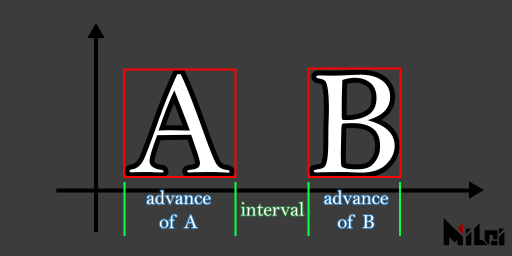## coord

coord() draws the current Paintbrush coordinate.coord.lua

version3()

move(100,100)
coord()

move(150,0)
coord()

rotate(d2r(30))
move(50,0)
scale(2,1)
coord()


## grid

grid() draws a grid with many 100x100px squares.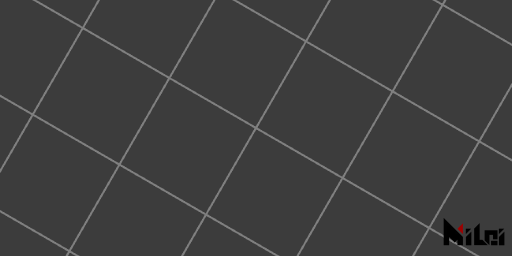grid.lua

version3()

move(width/2,height/2)
rotate(d2r(30))
grid()


## setPoly

setPoly(obj) analyzes obj only, use poly() to draw the previous set obj to scene.

• It will be efficient in the case you draw the same obj for many times.

## background

background(brightness),background(r,g,b),background(r,g,b,a) draws a pure color rectangle to scene.

• Notes: This function overrides all shapes you drew before.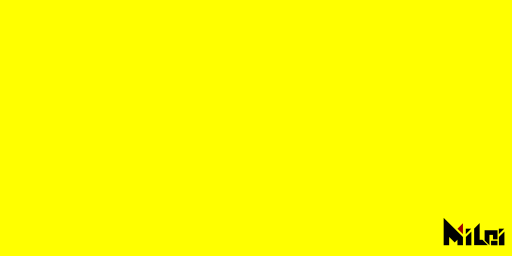background.lua

version3()

background(1,1,0)


## in2out

1. in2out(id) set the id texture to the scene.
2. in2out() equals to in2out(INPUT)

id range: PARAM0~PARAM9 or INPUT.

## dim2

dim2() set the scene to 2D mode. The 2D mode is on by default, you usually no need to call it.

In PixelsWorld, a 2D scene means a 3D scene without depth test and perspective.

## dim3

dim3() set the scene to 3D mode. Call it immediately after version3().

Note: Use viewSpace to change the far plane dimension if your layer size is not equal to the comp size. Otherwise, the shapes in 3D mode will be rendered to an unexpected position.

## perspective

perspective() set the scene to perspective mode, everything looks small in the distance and big on the contrary. Use viewSpace to change the camera information. Use lookAt to set the location of the camera.

Perspective mode is on by default after calling dim3().

## noPerspective

noPerspective() set the scene to orthogonal mode.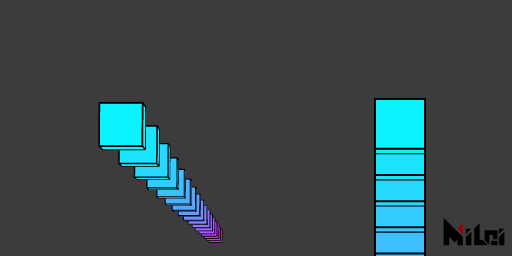noPerspective.lua

version3()

dim3()
n = 20
move(100,100,0)
beginGroup()
rotateX(d2r(85))
for i=1,n do
move(0,300,0)
fill(i/n,1-i/n,1)
cube(50)
end
endGroup()

move(300,0,0)
noPerspective()
beginGroup()
rotateX(d2r(85))
for i=1,n do
move(0,300,0)
fill(i/n,1-i/n,1)
cube(50)
end
endGroup()


## fill

1. fill() turns on fill mode.
2. fill(brightness),fill(r,g,b),fill(r,g,b,a) turns on fill mode and set fill color.
• Note: If you set Alpha smaller than 1, render far object first, or you will get wrong render results due to the depth test. (This is a feature of OpenGL render)

## noFill

noFill() turns off the fill mode.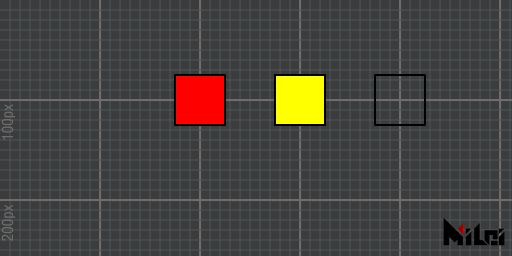fill.lua

version3()

move(200,100,0)
fill(1,0,0)
rect(50)

move(100,0,0)
fill(1,1,0)
rect(50)

move(100,0,0)
noFill()
rect(50)


## stroke

1. stroke() turns on the stroke mode.
2. stroke(brightness),stroke(r,g,b),stroke(r,g,b,a) turns on stroke mode and set the stroke color.

## noStroke

noStroke() turns off stroke mode.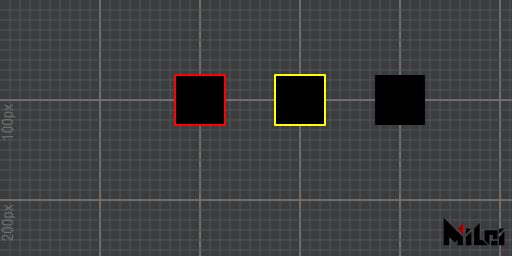stroke.lua

version3()

fill(0)
move(200,100,0)
stroke(1,0,0)
rect(50)

move(100,0,0)
stroke(1,1,0)
rect(50)

move(100,0,0)
noStroke()
rect(50)


## dot

1. dot() turns on the dot mode.
2. dot(brightness),dot(r,g,b),dot(r,g,b,a) turns on the dot mode and set the dot color.

## noDot

noDot() turns off the dot mode.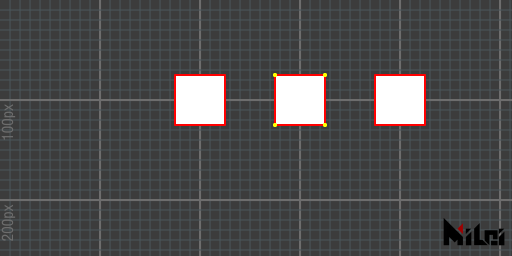dot.lua

version3()

fill(1)
move(200,100,0)
stroke(1,0,0)
rect(50)

move(100,0,0)
dot(1,1,0)
rect(50)

move(100,0,0)
noDot()
rect(50)


## wireframe

wireframe() turns on the wireframe mode.

## noWireframe

noWireframe() turns off the wireframe mode.wireframe.lua

version3()
n=8
dim3()

move(width/2, height/2)
for i=1,n do
if i>n//2 then wireframe()
else noWireframe() end
beginGroup()
rotateZ(d2r(i*360/n))
move(100,0,0)
fill(i/n,1-i/n,1)
ball(30,1)
endGroup()
end


## blendAlpha

blendAlpha() turns on the alpha blending.

## noBlendAlpha

noBlendAlpha turns off the alpha blending.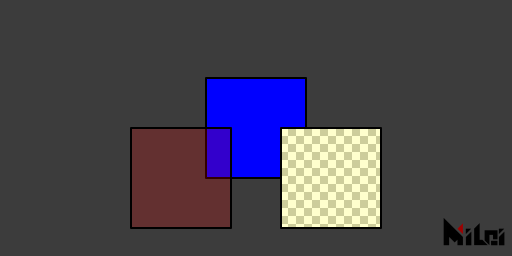blendAlpha.lua

version3()
castTex(OUTPUT,INPUT)

move(200,100,0)
fill(1,0,0,0.2)
rect(80)

move(100,0,0)
noBlendAlpha()
fill(1,0,0,0.2)
rect(80)


## back

back() If the fill alpha is smaller than 1, back mode shows the backside of an obj. Off by default.

## noBack

noBack() turn off back mode.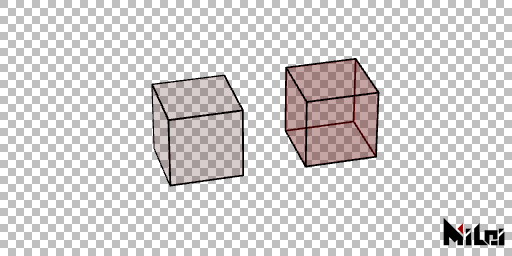back.lua

version3()
dim3()
fill(1,0,0,0.2)

rotateX(d2r(30))
rotateY(d2r(-15))
move(200,180,0)
cube(80)

move(150,0,0)
back()
cube(80)


## pure

pure() use pure color to render. On by default.

anime, phong, pure are 3 dependent mode, turn on one may turn off the other two.

## phong

1. phong(ambient,diffuse,specular,specularPower) turns on the phong render mode, and set the ambient strength to ambient, diffuse strength to diffuse, specular strength to specular, specular damping power to specularPower.
2. phong() only turn on the phong render mode, it doesn't change the configs.
• By default, there is no light in the scene, call getLight(), ambientLight(), parallelLight(), pointLight to add lights.
• If you are sure there are lights in your scene but the obj is black, call normal to check if the normal is right.
• Default settings: ambient:1,diffuse:1,specular:1,specularPower:1.
• anime, phong, pure are 3 dependent mode, turn on one may turn off the other two.
• Call dim3() before calling this function.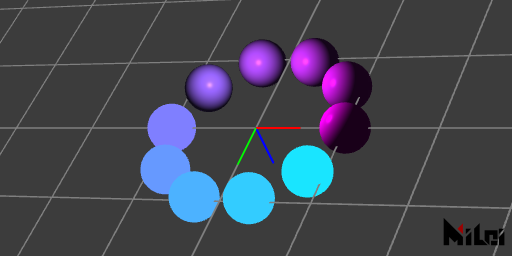phong.lua

version3()
dim3()

move(width/2,height/2,0)

grid()
coord()

n=10

beginGroup()
move(0,0,-100)
pointLight()
endGroup()

for i=1,n do
beginGroup()
rotateZ(d2r(i*360/n))
move(100,0,0)
fill(i/n,1-i/n,1)
if(i<=n//2) then pure()
else phong() end
ball(25)
endGroup()
end


## anime

1. anime(ambient,diffuse,specular,specularPower,diffuseThreshold,specularThreshold)
2. anime() turns on the anime render mode.
• anime render mode is based on phong, hence the first 4 arguments ambient,diffuse,specular,specularPower are same with phong render mode. diffuseThreshold configs the diffusion threshold, if diffuse lightnees in a pixel is bigger than threshold, it will be white. Otherwise it will be dark. specularThreshold is threshold of specular.
• The anime render mode smooth the light-dark border when you turn on the smooth settings in plugin panel.
• Default settings: ambient:1,diffuse:1,specular:1,specularPower:1,diffuseThreshold:0.5,specularThreshold:0.8 anime, phong, pure are 3 dependent mode, turn on one may turn off the other two.
• Call dim3() before calling this function.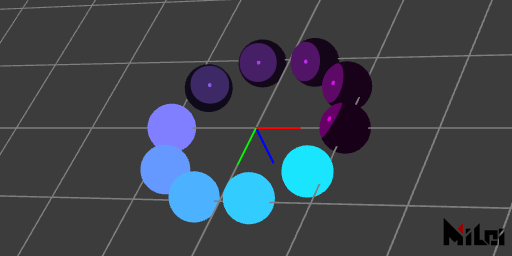anime.lua

version3()
dim3()

move(width/2,height/2,0)

grid()
coord()

n=10

beginGroup()
move(0,0,-100)
pointLight()
endGroup()

for i=1,n do
beginGroup()
rotateZ(d2r(i*360/n))
move(100,0,0)
fill(i/n,1-i/n,1)
if(i<=n//2) then pure()
else anime() end
ball(25)
endGroup()
end


## rgba

rgba()RGBA output mode, on by default.

• rgba, depth, normal are 3 dependent mode, turn on one may turn off the other two.
• You can use phong(),anime() in this output mode.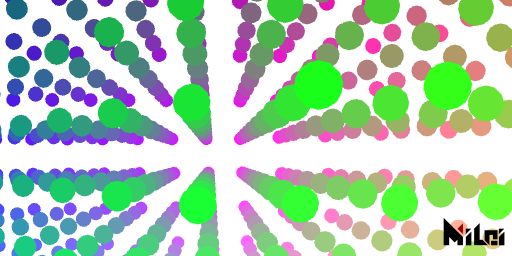rgba.lua

version3()
dim3()
background(1)
move(width/2,height/2,0)

n=10

beginGroup()
move(0,0,-100)
pointLight()
endGroup()

rgba()
-- depth()
-- normal()

noStroke()
for x=1,n do
for y=1,n do
for z=1,n do
beginGroup()
fill(x/n,y/n,z/n)
move(map(x,1,n,-n/2,n/2)*50,map(y,1,n,-n/2,n/2)*50,map(z,1,n,-n/2,n/2)*200)
ball(10,2)
endGroup()
end
end
end


## depth

depth(blackDistance, whiteDistance) depth output mode. Set pixels at distance blackDistance to black, pixels at distance whiteDistance to white. If they are the same, PixelsWorld sets the pixels nearer than the value you set to black, otherwise white.

• rgba, depth, normal are 3 dependent modes, turn on one may turn off the other two.
• phong(),anime() will be ignored in this output mode.
• Call dim3() before calling this function.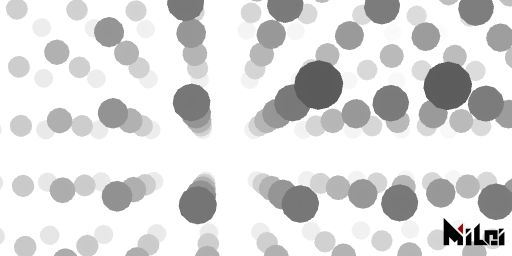depth.lua

version3()
dim3()
background(1)
move(width/2,height/2,0)

n=10

beginGroup()
move(0,0,-100)
pointLight()
endGroup()

-- rgba()
depth()
-- normal()

noStroke()
for x=1,n do
for y=1,n do
for z=1,n do
beginGroup()
fill(x/n,y/n,z/n)
move(map(x,1,n,-n/2,n/2)*50,map(y,1,n,-n/2,n/2)*50,map(z,1,n,-n/2,n/2)*200)
ball(10,2)
endGroup()
end
end
end


## normal

1. normal(faceToCamera, normalize) turns the normal output mode on, and configs faceToCamera and normalize.
2. normal(faceToCamera) turns the normal output mode on, and configs faceToCamera.
3. normal() only turns the normal output mode on.
• faceToCamera is a boolean, true means the normals are calculated based on camera location. false means the normals are calculated based on the global coordinate.
• normalize is a boolean.
• Default settings: faceToCamera:true, normalize:true.
• rgba, depth, normal are 3 dependent modes, turn on one may turn off the other two.
• phong(),anime() will be ignored in this output mode.
• Call dim3() before calling this function.normal.lua

version3()
dim3()
background(.5,.5,1)
move(width/2,height/2,0)

n=10

beginGroup()
move(0,0,-100)
pointLight()
endGroup()

-- rgba()
-- depth()
normal()

noStroke()
for x=1,n do
for y=1,n do
for z=1,n do
beginGroup()
fill(x/n,y/n,z/n)
move(map(x,1,n,-n/2,n/2)*50,map(y,1,n,-n/2,n/2)*50,map(z,1,n,-n/2,n/2)*200)
ball(10,2)
endGroup()
end
end
end


## setDepth

setDepth(id,blackDistance,whiteDistance) reads the red channel of texture id, maps color basing on blackDistance,whiteDistance to the depth test buffer in the scene.

• The depth sequence from other 3DCG software can be loaded into PixelsWorld through this function. Namely, the shapes can interact with the color sequence rendered from other 3DCG software.
• Call dim3() before calling this function.
• Valid texture id: INPUT,PARAM0~PARAM9

## ambientLight

1. ambientLight(r,b,g,intensity)
2. ambientLight() equals to ambientLight(1,1,1,1)
3. ambientLight(brightness) equals to ambientLight(brightness,brightness,brightness,1)
4. ambientLight(brightness,intensity) equals to ambientLight(brightness,brightness,brightness,intensity)
5. ambientLight(r,g,b) equals to ambientLight(r,b,g,1)
• Adds ambient light to the scene. Valid to all objs.
• Objs are lit up by this kind of light even if they have wrong normals.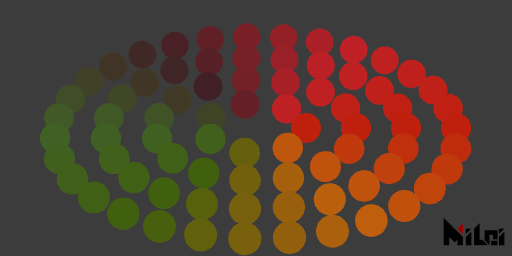al.lua

version3()
dim3()
move(width/2,height/2,0)

n=4

phong()

ambientLight(1,0.5,0.2,10)

noStroke()

for r=1,n do
local ra = r*50
local cn = math.floor(ra*TPI/40)
for i=1,cn do
beginGroup()
rotateZ(d2r(i/cn*360))
fill(hsl2rgb(i/cn,0.5,0.5))
move(ra,0,0)
ball(15,3)
endGroup()
end
end


## pointLight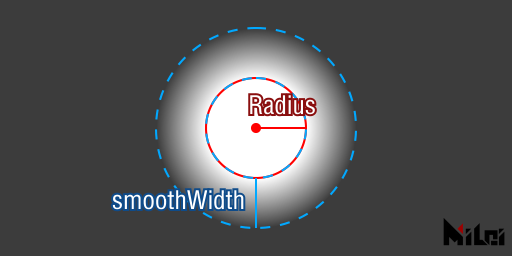1. pointLight(r,g,b,intensity,radius,smoothWidth)
2. pointLight() equals to pointLight(1,1,1,1,1000,1000)
3. pointLight(brightness,intensity) equals to pointLight(brightness,brightness,brightness,intensity,1000,1000)
4. pointLight(r,g,b) equals to pointLight(r,g,b,1,1000,1000)
5. pointLight(r,g,b,intensity) equals to pointLight(r,g,b,intensity,1000,1000)
6. pointLight(r,g,b,intensity,radiusAndSmoothWidth) equals to pointLight(r,g,b,intensity,radiusAndSmoothWidth,radiusAndSmoothWidth)
• Adds a point light in the current Paintbrush coordinate.
• This light is affected by objs' normals. The specular and diffuse will be failed if the normals are inward, but the ambient brightness of point light still lights up the objs.
• radius is range radius of point light. Range from radius to radius+smoothWidth, the brightness damps.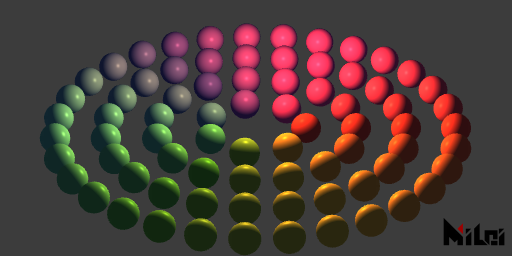pl.lua

version3()
dim3()
move(width/2,height/2,0)

n=4

phong()

ambientLight(0.2,0.5,1,2)

beginGroup()
move(0,0,-100)
pointLight(1,0.5,0.2,2,500,100)
endGroup()

noStroke()

for r=1,n do
local ra = r*50
local cn = math.floor(ra*TPI/40)
for i=1,cn do
beginGroup()
rotateZ(d2r(i/cn*360))
fill(hsl2rgb(i/cn,0.5,0.5))
move(ra,0,0)
ball(15,3)
endGroup()
end
end


## parallelLight

1. parallelLight(r,g,b,intensity,tx,ty,tz)
• Adds a parallel light with direction vector (tx,ty,tz).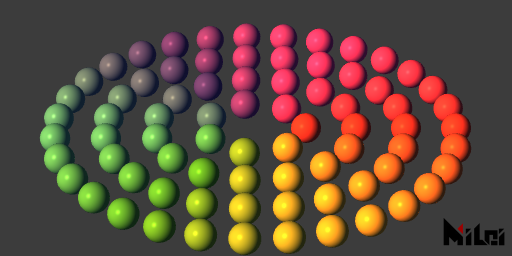pll.lua

version3()
dim3()
move(width/2,height/2,0)

n=4

phong()

ambientLight(0.2,0.5,1,2)
parallelLight(1,0.5,0.2,2,1,-1,1)
noStroke()

for r=1,n do
local ra = r*50
local cn = math.floor(ra*TPI/40)
for i=1,cn do
beginGroup()
rotateZ(d2r(i/cn*360))
fill(hsl2rgb(i/cn,0.5,0.5))
move(ra,0,0)
ball(15,3)
endGroup()
end
end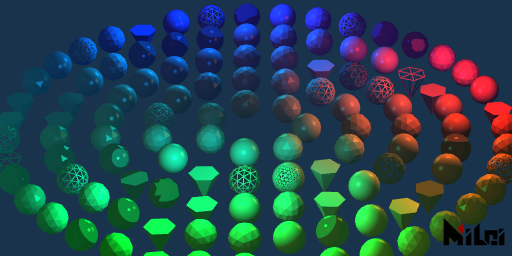light_effects.lua

version3()
dim3()
background(0.1,0.2,0.3)
move(width/2,height/2,0)
math.randomseed(1)
n=5

ambientLight(0.2,0.5,1,1)
parallelLight(1,0.5,0.2,0.1,1,-1,1)

beginGroup()
move(200,0,-100)
pointLight(1,0.5,0.2,1,200,100)
endGroup()

beginGroup()
move(-30,200,-100)
pointLight(0,1,1,1,200,100)
endGroup()

beginGroup()
move(-30,-200,-100)
pointLight(0,1,1,1,200,100)
endGroup()

noStroke()

for r=1,n do
local ra = r*50
local cn = math.floor(ra*TPI/40)
for i=1,cn do
beginGroup()
rotateZ(d2r(i/cn*360))
fill(hsl2rgb(i/cn,r/n,0.6))
move(ra,0,0)

if math.random() < .15 then
wireframe()
else noWireframe() end

if math.random() < .15 then
anime()
else phong() end

if math.random() <.8 then
if math.random() < .3 then
ball(15,3)
else ball(15,2) end
else cone(15,30,6) end

endGroup()
end
end


## clearLight

clearLight() removes all lights in the scene.

## getLight

1. getLight(matchName)
2. getLight() equals to getLight("*")
• Gets lights that match the name matchName.
• matchName rules：If matchName doesn't end up with character "*", it searches one Ae lights that its name is matchName, otherwise, it includes all Ae lights that begin with matchName.
• Supported Ae lights: ambient,point,parallel

## aeCamera

aeCamera() sets the Ae activated camera to the scene camera of PixelsWorld.

## lookAt

1. lookAt(eyePosX,eyePosY,eyePosZ,objPosX,objPosY,objPosZ,upVecX,upVecY,upVecZ) sets the location and orientation of the current scene camera.
2. lookAt(eyePosX,eyePosY,eyePosZ,objPosX,objPosY,objPosZ) equals to lookAt(eyePosX,eyePosY,eyePosZ,objPosX,objPosY,objPosZ,0,-1,0)
• eyePos is the location of your eyes, objPos is the location of the object you are looking at,upVec the direction the top of your head pointing to.
• Note: The Y-axis in Ae is downward by default, usually set the upVec to (0,-1,0) is enough.
• eyePos and objPos cannot be too close. (should be bigger than 1e-7).
• upVec cannot be parallel to your sight.
• The length of upVec cannot be too small.

## viewSpace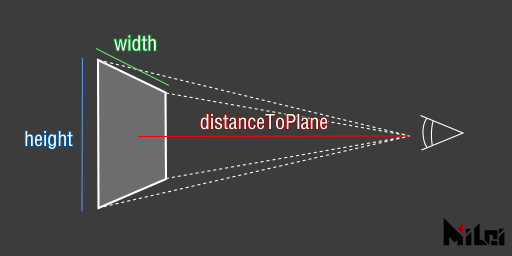1. viewSpace(width,height,distanceToPlane,farLevel)
2. viewSpace(width,height,distanceToPlane) equals to viewSpace(width,height,distanceToPlane,4)
• width and height is the dimension of the far plane.
• The perpendicular distance from the camera to the camera's far plane is distanceToPlane
• farLevel * distanceToPlane is the clip plane distance. Objs that farther than this distance will be clipped out. Normally it is enough to leave the farLevel 4, set it to a higher number if your scene is pretty vast. Note that if the farLevel is too high, the depth test precision of near objs may decline.

## strokeWidth

strokeWidth(width)

Default: 2

## strokeDivision

strokeDivision(level)

Default: 3

## strokeGlobal

strokeGlobal() draw lines globally. Lines will no longer be squeezed by scale function.

• Default: local

## strokeLocal

strokeLocal() draw lines locally. Lines will be squeezed by scale function.

• Default: local

dotRadius(radius)

• Default: 2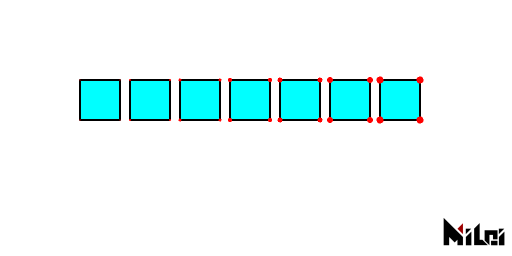version3()
background(1)
fill(0,1,1)
dot(1,0,0)
move(100,100)
for i=1,7 do
rect(40)
move(50,0)
end


## dotDivision

dotDivision(level)

• Default: 3
• Maximum: 7dotDivision.lua

version3()
background(1)
fill(0,1,1)
dot(1,0,0)
move(100,100)
for i=0,4 do
dotDivision(i)
rect(40)
move(70,0)
end


## dotGlobal

dotGlobal() Draw points globally. Points will no longer be squeezed by scale function.

• Default: local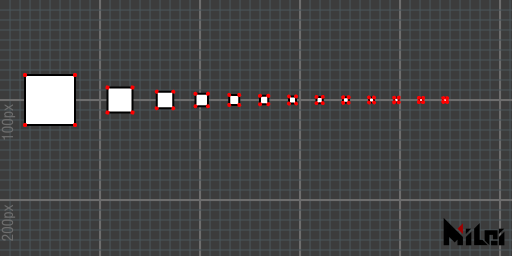dotGlobal.lua

version3()

dot(1,0,0)
dotGlobal()

move(50,100)

beginGroup()
for i=1,13 do
beginGroup()
scale(1/i)
rect(50)
endGroup()
move(50/i+20,0)
end
endGroup()


## dotLocal

dotLocal() Draw points locally. Points will be squeezed by scale function.

• Default: local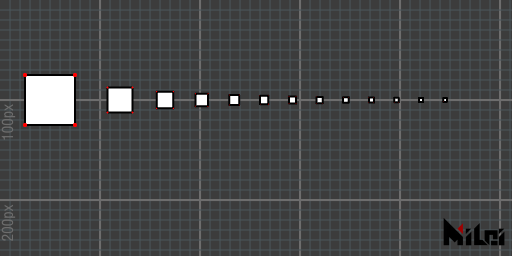dotLocal.lua

version3()

dot(1,0,0)
dotLocal()

move(50,100)

beginGroup()
for i=1,13 do
beginGroup()
scale(1/i)
rect(50)
endGroup()
move(50/i+20,0)
end
endGroup()


## smooth

smooth() Smooth render mode.

Default: on The smooth strength can be changed in the plugin panel.

## noSmooth

noSmooth() Pixel art render mode.

The priority of this function is higher than the settings in the plugin panel.

## r2d

r2d(degrees) radians to degrees, return degrees.

## d2r

d2r(radians) degrees to radians, return radians

## map

map(value,in1,in2,out1,out2) maps value value from range in1~in2 to range out1~out2.

If in1 equals to in2, if value<in1 this function returns out1, otherwise out2.

## clamp

clamp(value,lower,upper)clamps value into range [lower,upper], returns clamped value.

• New in v3.2.0
• Namely, if value is between lower and upper, this function returns value; If value is less than lower, it returns lower; If value is greater than upper, it returns upper.

## step

step(value,threshold) returns 0 if value<threshold, otherwise, returns 1.

• New in v3.2.0

## smoothStep

smoothStep(value,lower,upper) returns 0 if value<lower, returns 1 if value>higher1, otherwise, returns the smooth interpolation between lower and higher basing on value.

Formula of interpolation:

$t := \frac{x - lower}{upper - lower}$

$Result:=t^2(3 - 2t)$

• New in v3.2.0

## bezier

bezier(t,p0,p1,...,pn) returns the n times bezier interpolation basing on p0,p1,...,pn.

The formula of interpolation:

$Result := \sum_{k=0}^n{C_n^k\cdot (1-t)^{n-k}\cdot t^k\cdot p_k}$

$C_n^k:=\frac{n!}{(n-k)!k!}$

• New in v3.2.0
• The maximum of n is 66.

## Color conversion

xxx2xxx allows the following color conversion: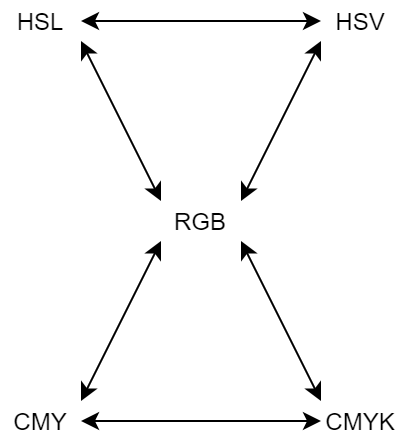For example, if you want to convert a hsl color to rgb format, then you need to call the function hsl2rgb, both the number of input and output parameters are 3.

All conversions are done in the range 0~1.

• New in v3.2.0color_convert.lua

version3()

dim3()
strokeWidth(0.5)
stroke(0)

move(width/2,height/2,0)
for x = -5,5 do
for y =-5,5 do
for z=-5,5 do
beginGroup()
move(x*15,y*15,z*15)
fill(cmy2rgb(x/10+.5,y/10+.5,z/10+.5))
cube(12)
endGroup()
end
end
end


## utf8ToLocal

utf8ToLocal(str) unicode string to local string.

Call this function to translate paths while you are handling Lua's io module.

## localToUtf8

localToUtf8(str)local string to unicode string

## getGLInfo

getGLInfo() gets the information of the current graphics card.

## getDrawRecord

1. getDrawRecord(needStringFormat) gets current draw records. needStringFormat is a boolean, when true, returns a string, otherwise, returns a Lua table.
2. getDrawRecord() equals to getDrawRecord(true)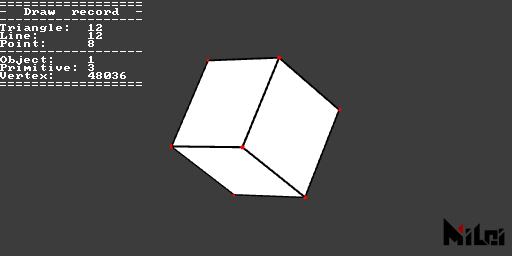printDrawRecord.lua

version3()
move(width/2,height/2)
dim3()
cube()
println(getDrawRecord());


## getStatus

1. getStatus(needStringFormat)gets the current Paintbrush status. needStringFormat is a boolean, when true, returns a string, otherwise, returns a Lua table.
2. getStatus() equals to getStatus(true)

## getAudio

Make sure you have PixelsWorld v3.4.0+

1. getAudio([startTime,duration[,id[,sampleRate,startFrequency,endFrequency[,resolution]]]]) fetches the audio data. Returns 6 tables. The first 2 are wave sample tables(Left and Right), the next 2 are fft result tables(Left and Right), the last 2 are spectrum tables(Left and Right).
• Left wave sample range: (-1~1)
• Right wave sample range: (-1~1)
• Left FFT range: (0~infinity)
• Right FFT range: (0~infinity)
• Left spectrum sample range: (0~infinity)
• Right spectrum sample range: (0~infinity)

waveInfo.lua

version3()
castTex(OUTPUT,INPUT)

local wl,wr,ftl,ftr,specl,specr = getAudio()

local nm = math.floor(height/8);

for i=1,nm do
local wid =math.max(math.floor(i/nm*#wl),1)
local fid = math.max(math.floor(i/nm*#specl),1)
print(string.format("%8.5f",wl[wid]),wl[wid]*4,0,-wl[wid]*4)
print("  < L  R >  ",1,0,0,0)
print(string.format("%8.5f",wr[wid]),wr[wid]*4,0,-wr[wid]*4)
print("      <   Wave  FFT   >      ",0.5,0.5,0.5)
print(string.format("%8.5f",specl[fid]),specl[fid],0,0)
print("  < L  R >  ",1,0,0,0)
print(string.format("%8.5f",specr[fid]),0,0,specr[fid])
println("");
end


## saveString

saveString(utf8_path,string) saves string to local path.

loadString(utf8_path) reads local txt file then returns string.

## getColor

getColor(id,x,y) get pixel color at location (x,y) of texture id. Returns r,g,b,a 4 doubles. getColor(x,y) equals to getColor(INPUT,x,y)

• It is highly efficient to call getColor before any draw function(Such as immediately after version3()). Otherwise, it is very low efficient since it queries pixels from the graphic card.
• Valid id: INPUT,OUTPUT,PARAM0~PARAM9

## setColor

setColor(x,y,r,g,b,a) set the pixel at location (x,y) of texture OUTPUT.

• It is highly efficient to call setColor before any draw function

## getSize

getSize(id) returns the size of texture id. (Two doubles, width, and height)

Due to the Ae's downsample (1/2,1/4) mechanism, the size you get would variating 0~4px. But this kind of variating doesn't change with time. The size is promised to be accurate in full resolution(without downsample).

shadertoy(code) runs code from shadertoy.com.

• Not all code on shadertoy.com is supported.

## glsl

glsl(code) runs fragment stage shader code.

## cmd

cmd(code) runs cmd code.

This function is equivalent to the ISO C function system. It passes a command to be executed by an operating system shell. Its first result is true if the command terminated successfully, or nil otherwise. After this first result the function returns a string plus a number, as follows:

• "exit": the command terminated normally; the following number is the exit status of the command.
• "signal": the command was terminated by a signal; the following number is the signal that terminated the command.

## lua

lua(code) runs lua code.

## runFile

runFile(utf8_path) loads local lua code and run.

No need to call utf8ToLocal since it supports utf8.

## txt

txt(utf8_path) loads local text file. Returns string.

No need to call utf8ToLocal since it supports utf8.